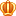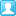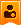##帐号 自动登录 找回密码 密码 立即注册
 搜索

# [已解决]请问这里最后一行代码为啥没有调动property里面的doc?发表于 2021-9-14 15:55:23 | 显示全部楼层 |阅读模式
10鱼币
 class Foo:     def __init__(self,num):         self.age = num     @property     def get(self):         return self.age     @get.setter     def get(self,num1):         self.age = num1 class temp:     def __init__(self,time):         self.count = time     def get(self):         return self.count     def set(self,time1):         self.count = time1     def delete(self):         del self.count         print ('已删除')     p = property(get,set,delete,'修饰符property的使用') #a = Foo(10) #print (a.get) #a.get = 20 #print (a.get) b = temp(5) print (b.p) b.p = 10 print (b.p) print (b.__dict__) del b.p print (b.__dict__) b.p.__doc__复制代码 最佳答案阿奇_o 2021-9-14 15:55:24 你用 类名.p.__doc__  就行了。。 In : class temp:    ...:     def __init__(self,time):    ...:         self.count = time    ...:     def get(self):    ...:         return self.count    ...:     def set(self,time1):    ...:         self.count = time1    ...:     def delete(self):    ...:         del self.count    ...:         print ('已删除')    ...:    ...:     p = property(get,set,delete,'修饰符property的使用')    ...: In : t = temp() In : t = temp(60) In : t.p Out: 60 In : t.__doc__ In : temp.__doc__ In : temp.p.__doc__ Out: '修饰符property的使用'复制代码 # 至于为什么是这样子？ 我也不想去进一步了解，哈哈，反正这样的装饰器的__doc__特性，几乎用不上。更常用的是直接用@property .best_avatar img{width: 30px;height: 30px;border-radius: 30px;}

### 最佳答案发表于 2021-9-14 15:55:24 | 显示全部楼层    本楼为最佳答案
 .pct{min-height:100px;}你用 类名.p.__doc__  就行了。。 In : class temp:    ...:     def __init__(self,time):    ...:         self.count = time    ...:     def get(self):    ...:         return self.count    ...:     def set(self,time1):    ...:         self.count = time1    ...:     def delete(self):    ...:         del self.count    ...:         print ('已删除')    ...:    ...:     p = property(get,set,delete,'修饰符property的使用')    ...: In : t = temp() In : t = temp(60) In : t.p Out: 60 In : t.__doc__ In : temp.__doc__ In : temp.p.__doc__ Out: '修饰符property的使用'复制代码 # 至于为什么是这样子？ 我也不想去进一步了解，哈哈，反正这样的装饰器的__doc__特性，几乎用不上。更常用的是直接用@property发表于 2021-9-16 12:36:17 | 显示全部楼层
 b.p 对象都被你 del 删除了，再对该对象 __doc__ 肯定会报错的 把 b.p.__doc__ 代码放再 del b.p 前一行即可楼主| 发表于 2021-9-16 20:17:57 | 显示全部楼层
 Twilight6 发表于 2021-9-16 12:36 b.p 对象都被你 del 删除了，再对该对象 __doc__ 肯定会报错的 把 b.p.__doc__ 代码放再 del b.p 前一 ...class temp:     def __init__(self,time):         self.count = time     def get(self):         return self.count     def set(self,time1):         self.count = time1     def delete(self):         del self.count         print ('已删除')     p = property(get,set,delete,'修饰符property的使用') #a = Foo(10) #print (a.get) #a.get = 20 #print (a.get) b = temp(5) #print (b.p) #b.p = 10 #print (b.p) #print (b.__dict__) #del b.p #print (b.__dict__) print (b.p.__doc__)复制代码 我改成运行后返回： int([x]) -> integer int(x, base=10) -> integer Convert a number or string to an integer, or return 0 if no arguments are given.  If x is a number, return x.__int__().  For floating point numbers, this truncates towards zero. If x is not a number or if base is given, then x must be a string, bytes, or bytearray instance representing an integer literal in the given base.  The literal can be preceded by '+' or '-' and be surrounded by whitespace.  The base defaults to 10.  Valid bases are 0 and 2-36. Base 0 means to interpret the base from the string as an integer literal. >>> int('0b100', base=0) 4 没懂为啥会返回这个，我想让他返回property里面我输入的文本

 您需要登录后才可以回帖 登录 | 立即注册 本版积分规则 回帖后跳转到最后一页

GMT+8, 2021-9-26 18:29